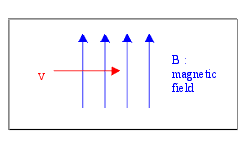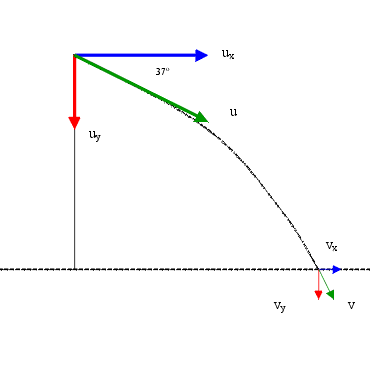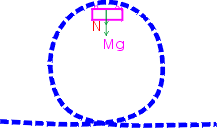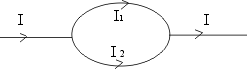Q
A singly ionized particle (q = 1.60 x 10-19 C) is moving at 1.9 x 104 m/s and moves perpendicular into a magnetic field of 1.0 x 10-3 T. If the particle moves in a radius of curvature of 0.40 m, determine the mass of the particle. Begin your solution with a statement about forces.

Ans
radius of curvature r = 0.4 m
magnetic field B = 1 x 10-3 T
charge = 1.6 x 10-19 C
velocity v = 1.9 x 104 m/sA force acts on the charged particle in a magnetic field which is given by F= qv x B. Direction of force is given by right hand screw rule. In this case it is perpendicular to the paper, outwards. A force perpendicular to the direction of motion can change only the direction of motion and therefore makes it move in a circular path. This force thus provides the centripetal force.

F =qv x B = qvBSin() = qvB = mv2/r

where m is the mass of the particle and r is radius of the path

m = Bqr/v = (1 x 10-3 x 1.6 x 10-19 x 0.4) / 1.9 x 104 = 0.34 x 10-26 kgQ A plane fires a bullet with an initial velocity of 100m/s at an angle of 37 degrees below the horizontal. The bullet lands two seconds later. In the questions that follow, you may neglect air resistance and assume that g = 10 m/s/s.

a. Find the horizontal (x) and the vertical (y) components of the bullet's initial velocity.

b. How far does the bullet travel in the horizontal direction before it lands? Show your work.

c. How high above the (level) ground was the plane when the bullet was fired? Show your work.

d. Find the magnitude of the velocity of the bullet right before it hits the ground. Show your work.

Ans au = 100 m/s
angle A = 37 degrees
g = 10 m/s2
t = 2 s
Horizontal component of bullets initial velocity = ux = u Cos 37 = 80 m/s
Vertical component of bullets initial velocity = uy = u Sin 37 = 60 m/s

Ans b

The acceleration in the horizontal direction = ax = 0
therefore distance traveled in the horizontal direction x = ux t
here ux has been found out to be 80 m/s
therefore x = 80 x 2 = 160 m

Ans c

Acceleration in the vertical direction = g = 10 m/s2
Distance traveled in the vertical direction

h = uyt + ½ ayt2 = uyt + ½ gt2

= 60 x 2 x ½ x 10 x 4 = 100 m

Ans d

vx = x (horizontal) component of final velocity

vy = y (vertical) component of final velocity

vx = ux + ax t = 80 m/s as acceleration in horizontal direction is zero

vy = uy + gt = 60 + 10x2 = 80 m/s

v = final velocity =(vx2 + vy2)1/2 = (802 + 802)1/2 =113.14 m/s

Angle of v = B = tan-1vy/vx = tan-1 1 = 45 degrees

Q. Determine the kinetic energy of an electron that has a wavelength of 1m.

A. = λ h/p
h = 6.63 x 10 -34 Js
or p = h/λ = (6.63 x 10 -34Js)/1m
k = p2/2m = (6.63 x 10 -34)2 / 2 x 9.1 x 10 -31 = 2.4 x 10 -37 J
= (2.4 x 10 -37) / 1.6 x 10 -19 = 1.5 x 10 -18 eV

Q An observer looks at a source through a grating having 1000 slits per cm. If the wavelength is 550nm, how many images of the source can be seen? (Hint: For what order would theta be + or - 90 degrees)

A.

d = grating spacing
deviation angle = 30 degs
λ = wave length of light
n = spectral order
N = no of lines / cm = 1000
N= 1/d or d = 1/N = 1/103 cm = 10-3 cm = 10 -5 m
nλ = d Sin
A
λ = 550 nm = 550 x 10 -9 m.
Maximum number of images will be seen for greatest angle of deviation i.e.
A = + or - 90 degs
hence Sin
A = + or - 1
therefore the order numbers for the visible spectrum for the grating are limited to
n < = d / λ = 10 -5 / 550 x 10 -9 = 18.2
hence 18 complete orders will be observed, or 36 images;18 on each side of central maximum
(x-1)mo c2 = mo c2
{1 / (1 - v2 / c2) - 0.5} - 1 = 1
1 / (1 - v2 / c2) - 0.5 = 2
1 - v2 / c2 = 1/4
v = 0.866cQ. Draw a motion diagram and a free-body diagram for a roller coaster car at the top of a "loop-de-loop" (i.e., when the car is upside down). Do not ignore air resistance, and assume that the car is moving rapidly enough to remain in firm contact with the rails. In particular, explain why there is no outward force on the car.A. The forces on the roller coaster are ( in the absence of air friction and friction between rails and the car)

a. Weight mg downwards

b. Normal force N by the rails on the car, also downwards

From Newton's second law N + mg = (mv2 )/ r = centripetal force to execute the circle with velocity v

Min speed at the top = (rg)-2 where r is the radius of the loop

This speed is derived from conservation of energy. If air friction is taken into account, then v must be greater than (rg)-2 as part of the energy will be used to overcome friction.

Outward force or cetrifugal force is a pseudo force which needs to be taken into account if we describe the motion of the car on the roller coaster from a rotating frame which is non inertial and still use Newton's laws. As we are observing the roller coaster from the ground frame, which is inertial, there is no pseudo force.Q. A coil of diameter 3 m having 120 turns and resistance 8.0 m ohms is flat on a table, and a magnetic induction perpendicular to its plane changes at a steady rate from 0 to 0.8T. How much charge flowed through the coil during the time required for the field to change?

A Flux Ø = nAB

this implies that e = nAdB/dt =iR

which implies that nAdB = iRdt

Integrating both sides of the above equation with limits

q = nAB/R = nAx0.8/R

= (120 x pi x (1.5)2 x 0.8)/8 = 84.78 CoulombQ The ac generator in Fig below supplies 120 V (rms) at 60 Hz. With the switch open as in the diagram, the resulting current leads the generator emf by 20 degs. With the switch in position 1 the current lags the generator emf by 10 deg. When the switch is in position 2 the rms current is 2.0 A. find the values of R,L, and c.Ev = 120V , f=60 Hz , w=2pif = 360 radians

Current I leads by 20 degs

Phase is negative, Xl < Xc

Tan (-20deg)= (Xl - Xc)/R = -0.36 ---- (1)

Or (Xc -Xl)/R =0.36

I lags by 10 degs and phase is positive

Xl > Xc1

Tan 10 degs = 0.18 = (Xl - Xc1)/R ------(2)

Z=Xc - Xl = Ev /Iv = 120/2 =60 ---------(3)

Substituting 3 in 1

60/R=0.36

R=60/0.36 = 500/3 ohms

Equation 2 implies wL-1/2wC = 0.06 x 500/3 = 30

Equation 3 implies 1/wC -wL=60 ----------(a)

wL = 30 + 1/2wC ------(b)

substituting in a

1/wC -30 - 1/2wC =60

1/2wC = 90 -------------(c)

C=0.15 µF

Substituting c in b

wL=30 + 90

L= 1/3 H

Q Find the magnetic field at the center of the loop shown.B = (µo/4pi)(IL/r2)

B = 0

Since I1R1 = I2R2 or I1L1 = I2L2

Or B = (µo/4pir2)( I1L1 - I2L2) = 0

Q What is the back emf in a motor that draws 7.1 A from a 220 volt line if the field coils have a resistance of 200 ohms and are wound in parallel with an armature with a resistance of 3 ohms?

I=7.1 A , v = 220 V , R1 = 200 ohms, R2 = 3 ohms

Let E = back emf

I =(V-E)/R

1/R =1/3 +1/200 ie R = 600/203

E = V - IR = 220 - 21 = 199 V

Q. A horizontal rod 2 meters long is released in a region where there is a uniform horizontal magnetic induction of 0.6 Tesla perpendicular to the rod. How far has the rod fallen when the induced emf is 120V.

E=Blu

120 = 0.6 x 2x u

u = 100 m/s

initially u = o finally u =100 m/s

v2 - u2 =2as = 2gh

h= (1002 -0)/(2x10) =500 m